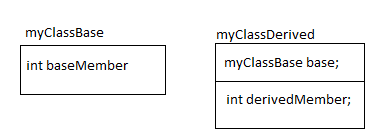# Inheritance

Let's consider these two classes -
```class myClassBase
{
public:
int baseMember;
myClassBase() { baseMember = 0; }
int baseMethod(int x) { return baseMember + x; }
};
class myClassDerived : public myClassBase
{
public:
int derivedMember;
myClassDerived() { derivedMember = 0; }
int derivedMethod(int x) { return derivedMember + x; }
};
```

The equivalent C code will be
```struct myClassBase
{
int baseMember;
};
struct myClassDerived
{
struct myClassBase base;
int derivedMember;
};
void myClassBase_ctor(struct myClassBase *this)
{
this->baseMember = 0;
}
int myClassBase_baseMethod(struct myClassBase *this, int x)
{
return this->baseMember + x;
}
void myClassDerived_ctor(struct myClassDerived *this)
{
myClassBase_ctor(this);
this->derivedMember = 0;
}
int myClassDerived_derivedMethod(struct myClassDerived *this, int x)
{
return this->derivedMember + x;
}
```

The relation between the classes can be described by a diagram as:Here are few points to understand this:
• The first member of the derived structure is of the type base.
• If the object of the derived is casted (using pointers) as the base member, it will point to the first member of the struct which is of type base struct.
• Defining the structure this way helps in implementing the polymorphism (the pointer of derived can be used as the pointer of the base).
• The constructor of the derived class first calls the base class constructor. This ensures that the base class is constructed first then the derived class.
• The constructor of the derived class can specify which constructor of the base class should be called. This is C++ language struct. If nothing is specified, the default constructor(with no parameter) is called.
• Constructor of the derived class is called from the code where the object is instantiated. The constructor of the derived class calls the constructor of its base.

Do you collaborate using whiteboard? Please try Lekh Board - An Intelligent Collaborate Whiteboard App